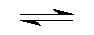## Acid - Base Indicators Methyl Red

by Aris Kaksis , Riga Stradin University RSU

Acid - Base indicators (also known as pH indicators) are substances which change colour with pH. They are usually weak acids or bases, which when dissolved in water dissociate slightly and form ions.
Consider an indicator which is a weak acid, with the protonated formula H2In2+. At equilibrium, the following equilibrium equation is established with its conjugate base neutral In:

H2In2+ (aq)      +       2 H2O (l)2 H3O+ (aq)     +    In(aq)
acid                                                                              conjugate base
(colour A)                                                                              (colour B)

The acid and its conjugate base have different colours. At low pH values the concentration of H3O+ is high and so the equilibrium position lies to the left. The equilibrium solution has the colour A. At high pH values, the concentration of H3O+ is low - the equilibrium position thus lies to the right and the equilibrium solution has colour B.
Methyl Red is an example of an indicator which establishes this type of equilibrium in aqueous solution:

yellow (Acid)                                                              red (Base)

Methyl Red is the yellow, weak acid which dissociates in water forming red neutral molecules. Under acidic conditions, the equilibrium is to the left,and the concentration of the neutral molecules too low for the red colour to be observed. However, under neutral and alkaline conditions, the equilibrium is to the right, and the concentration of theneutral molecules becomes sufficient for the red colour to be observed.
We can apply equilibrium law to indicator equilibria - in general for a weak acid indicator:

KIn = ( [H3O+]2·[In] / [H2In2+] ) eq

Kln is known as the indicator dissociation constant. The colour of the indicator turns from colour A to colour B or vice versa at its turning point. At this point:

[H2In2+]   =[In]

So from equation:

KIn =  [H3O+]2 = 10-10.2   ;     pKIn / 2 = 5.1 = pH = -log{SQRT( [H3O+]2 )}

The pH of the solution at its turning point is called the pKln and is the pH at which half of the indicator is in its acid form and the other half in the form of its conjugate base.

### Indicator Range

At a low pH, a weak acid indicator is almost entirely in the [H2In2+] form, the colour of which predominates. As the pH increases - the intensity of the colour of [H2In2+] decreases and the equilibrium is pushed to the right. Therefore the intensity of the colour of [In-] increases. Physiological condition for certainly distinct colour is assumed at ratio for conjugated base form:

[In] /  [H2In2+]   = 10  ; and for acid coloured form :   [In] /  [H2In2+]   = 1 / 10 = 0.1

-KIn = ([H3O+]2·[In] / [H2In2+])eq = 10-10.2 *10  ;  +KIn = ([H3O+]2·[In] / [H2In2+])eq = 10-10.2 *0.1

-pHIn = 9.2 / 2 =4.6                                          ;   +pHIn = 11.2 / 2 = 5.6

An indicator is most effective if the colour change is distinct and over a low pH range. For most indicators the range is within ±0.5 of the pKln value: - please see the table below for examples, to the right is a model of the acid form of each indicator - with the colour of the solution at the turning point. Number n of involved hydrogen protons H+ in equilibrium .

 Indicator return to index Colour pH colour      turning      point pH range Acid Base pKln / n -pH+pH Thymol Blue - 1st change red yellow 1.7 0.7 - 2.7 Methyl Orange red yellow 3.7 3.2 - 4.2 Bromocresol Green yellow blue 4.7 4.2 - 5.2 Methyl Red yellow red 5.1 4.6 - 5.6 Bromothymol Blue yellow blue 7.0 6.5 - 7.5 Phenol Red yellow red 7.9 7.4 - 8.4 Thymol Blue - 2nd change yellow blue 8.9 7.9 - 9.9 Phenolphthalein colourless pink 9.4 8.9 - 9.9

A Universal Indicator is a mixture of indicators which give a gradual change in colour over a wide pH range - the pH of a solution can be approximately identified when a few drops of universal indicator are mixed with the solution.

Indicators are used in titration solutions to signal the completion of the acid-base reaction.